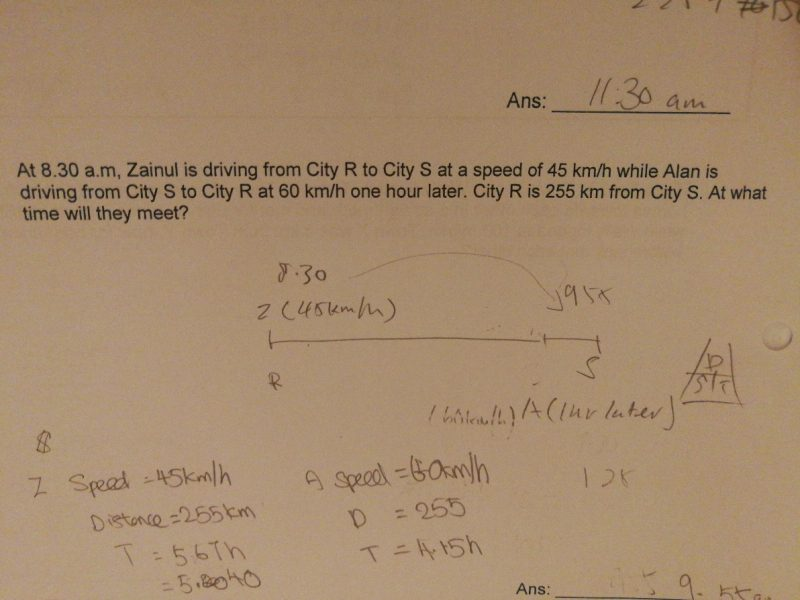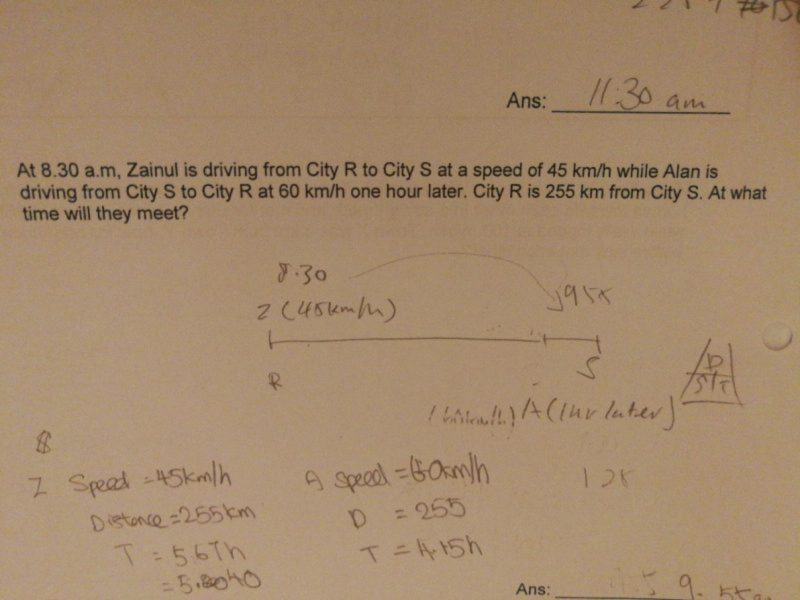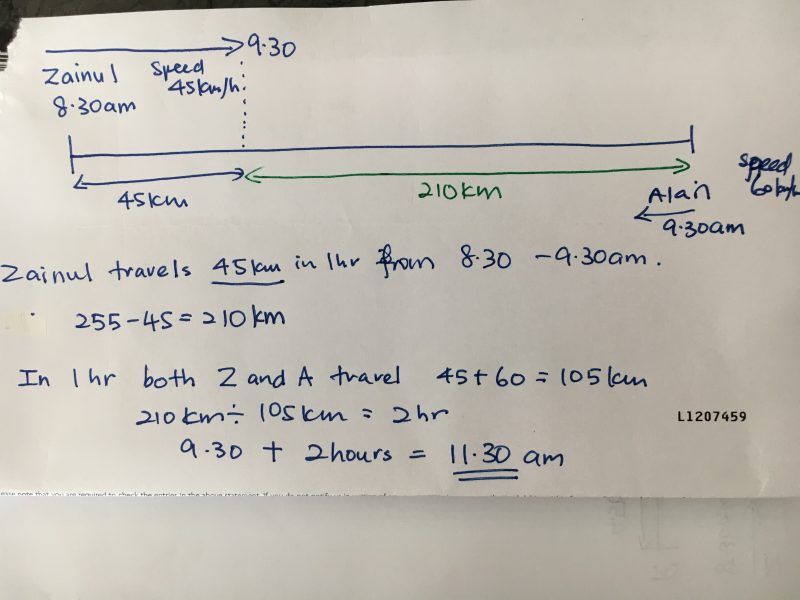# QuestionHi,

Can someone help on the above question?

Thank you.255km-(45km/h x 1h)=255km-45km=210km

210km/(45km/h+60km/h)=210km/105km/h=2h

2h+1h=3h

3h after 8.30 a.m. is 11.30 a.m.

0 Replies 0 Likes ✔Accepted Answer

255 – 45 = 210
210/(45 + 60) = 2
8.30 am ————– 9.30 am ——————— 2 hours ——————— 11.30 am

Ans : 11.30 am.

0 Replies 0 Likes0 Replies 0 Likes

This is similar to the one you posted previously,
https://www.kiasuparents.com/kiasu/question/62004-2/#post-62022

Instead of using 10u as the distance, the distance is 210km.
Zainul had a 1 hour head start, and base on his speed, he travelled 45km.
So the remaining distance is 255 – 45 = 210 km

Ratio of distance travelled when they meet
Z : A : Total => 45 : 60 : 105 => 90 : 120 : 210

Using Zainul distance and speed,
Time taken = 90/45 = 2 hours

Since the above ratio and calculation is based on 1 hours later, it’s actually 3 hours from 8.30am. The time they will meet is 11:30am ##

0 Replies 0 Likes